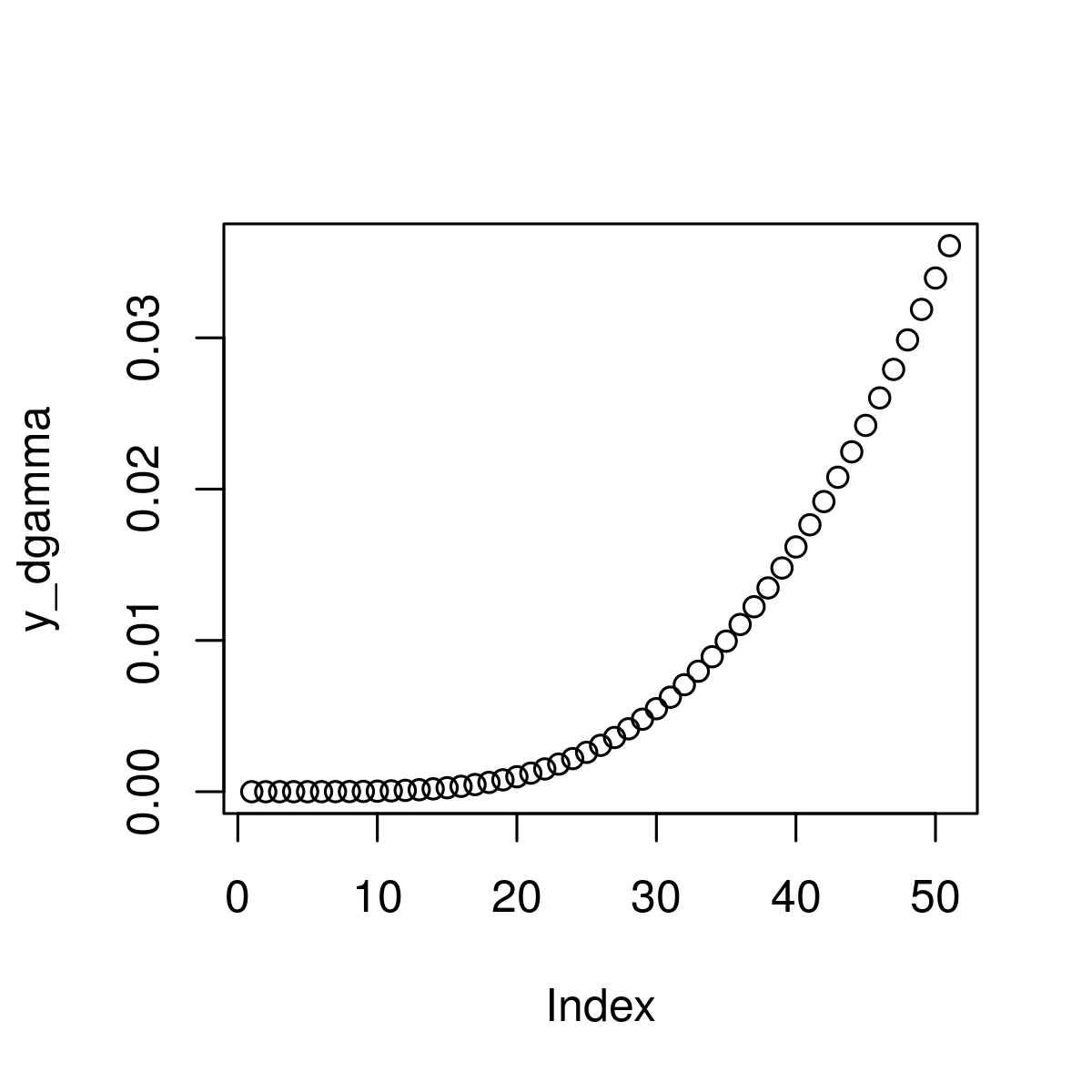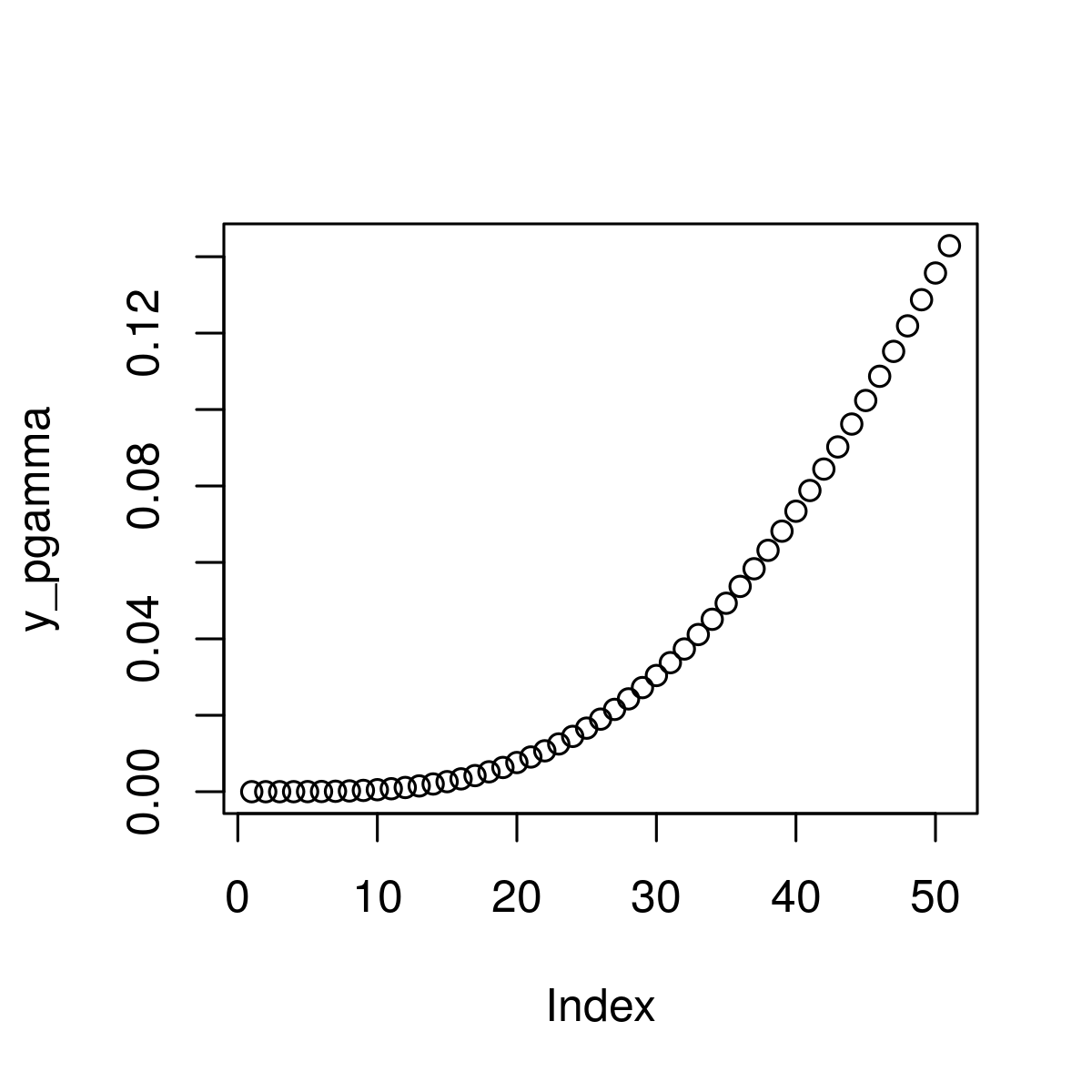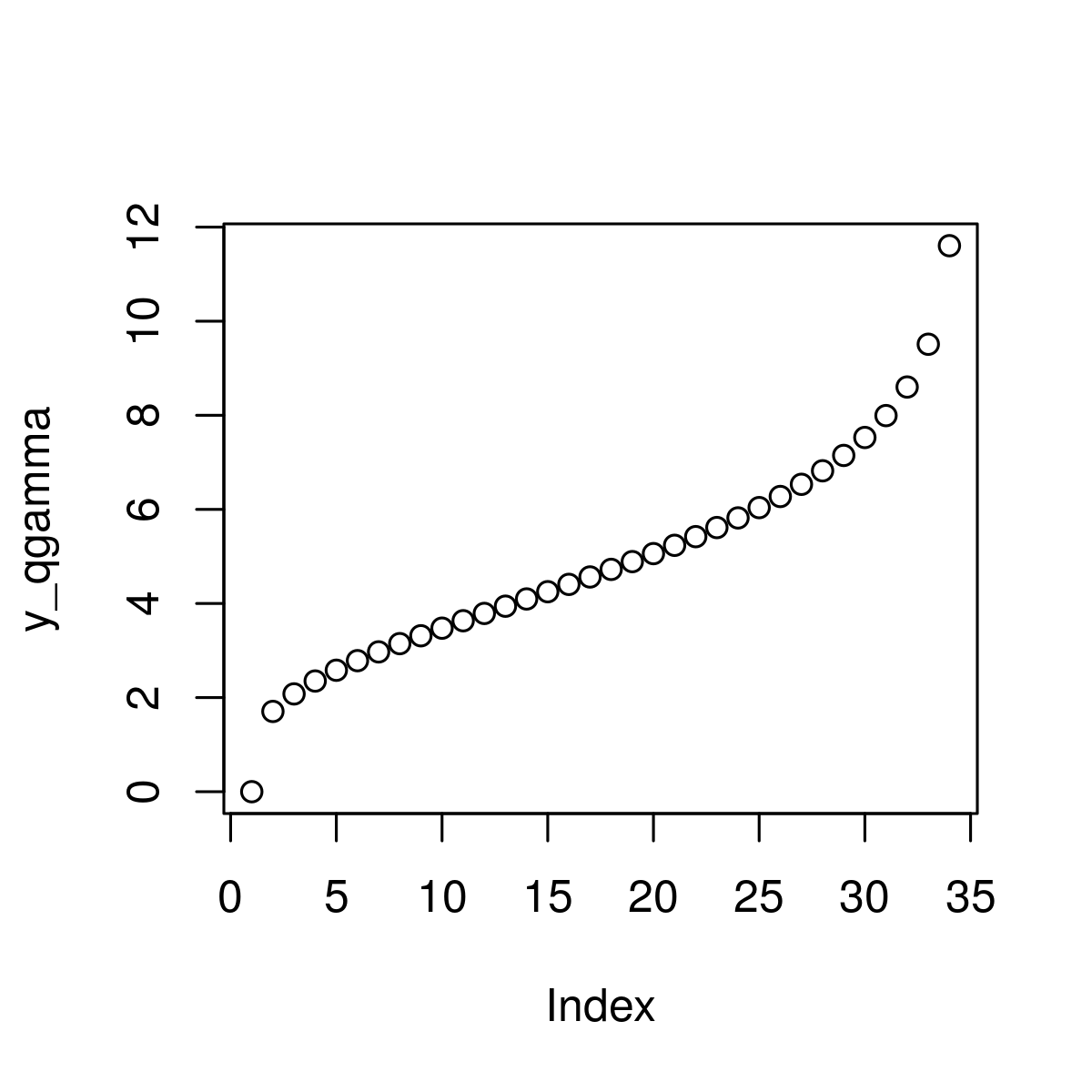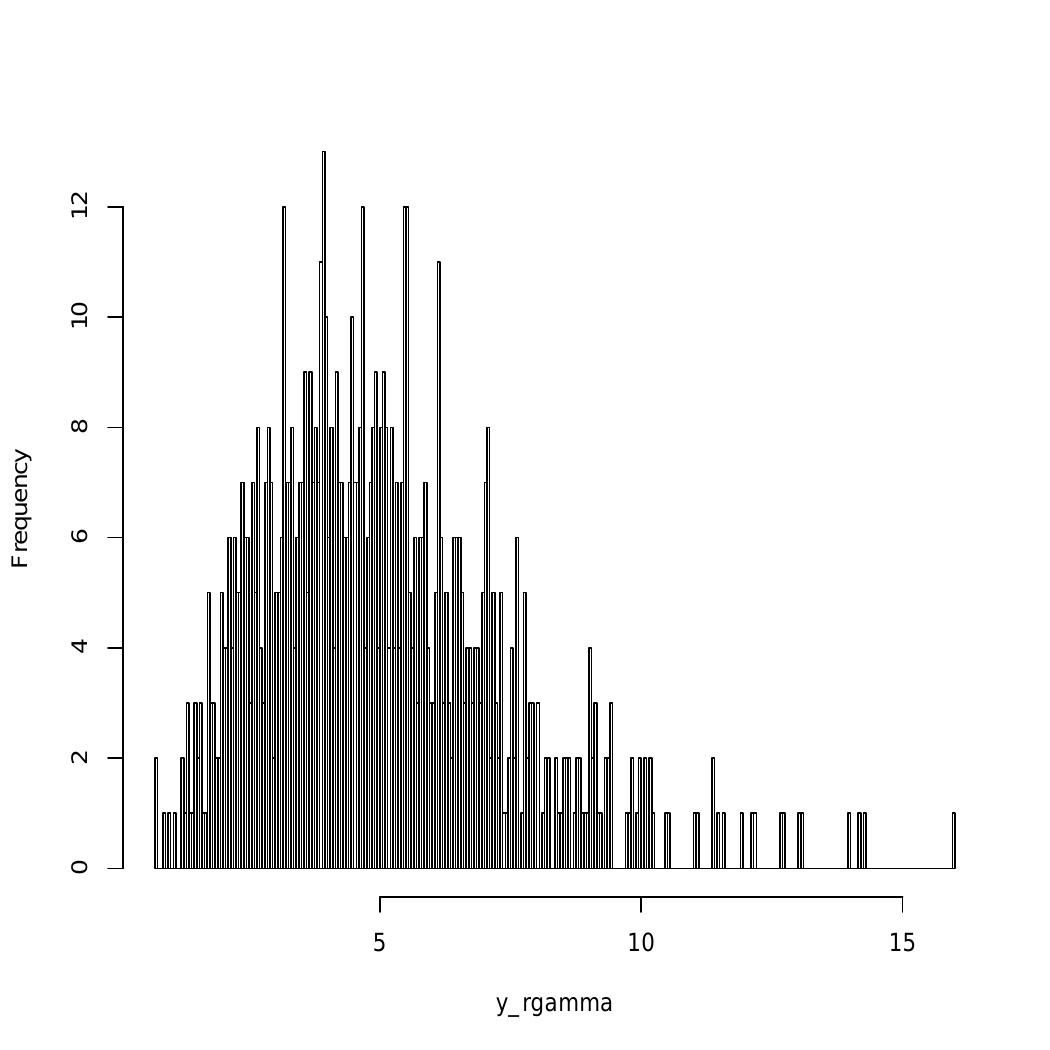Related Articles
Gamma Distribution in R Programming – dgamma(), pgamma(), qgamma(), and rgamma() Functions
• Last Updated : 30 Jun, 2020

The Gamma distribution in R Language is defined as a two-parameter family of continuous probability distributions which is used in exponential distribution, Erlang distribution, and chi-squared distribution. This article is the implementation of functions of gamma distribution.

#### dgamma() Function

`dgamma()` function is used to create gamma density plot which is basically used due to exponential and normal distributions factors.

Syntax:
dgamma(x_dgamma, shape)

Parameters:
x_dgamma: defines gamma function
shape: gamma density of input values

Returns: Plot dgamma values

Example :

 `# R program to plot gamma distribution`` ` `# Specify x-values for gamma function``x_dgamma <- ``seq``(0, 2, by = 0.04)   `` ` `# Apply dgamma function``y_dgamma <- ``dgamma``(x_dgamma, shape = 6) `` ` `# Plot dgamma values``plot``(y_dgamma)`

Output :#### pgamma() Function

`pgamma()` function is used in cumulative distribution function (CDF) of the gamma distribution.

Syntax:
pgamma(x_pgamma, shape)

Parameters:
x_pgamma: defines gamma function
shape: gamma density of input values

Returns: Plot pgamma values

Example:

 `# R program to plot gamma distribution`` ` `# Specify x-values for gamma function``x_pgamma <- ``seq``(0, 2, by = 0.04)   `` ` `# Apply pgamma function``y_pgamma <- ``pgamma``(x_pgamma, shape = 6) `` ` `# Plot pgamma values``plot``(y_pgamma)`

Output:#### qgamma() Function

It is known as gamma quantile function of the gamma distribution and used to plot qgamma distribution.

Syntax:
qgamma(x_qgamma, shape)

Parameters:
x_qgamma: defines gamma function
shape: gamma density of input values

Returns: Plot qgamma values with gamma density

Example :

 `# R program to plot gamma distribution`` ` `# Specify x-values for gamma function``x_qgamma <- ``seq``(0, 1, by = 0.03)   `` ` `# Apply qgamma function``y_qgamma <- ``qgamma``(x_qgamma, shape = 6) `` ` `# Plot qgamma values``plot``(y_qgamma)`

Output:#### rgamma() Function

This function is basically used for generating random number in gamma distribution.

Syntax:
rgamma(N, shape)

Parameters:
N: gamma distributed values
shape: gamma density of input values

Returns: Plot rgamma values with gamma density

Example :

 `# R program to plot gamma distribution`` ` `# Set seed for reproducibility``set.seed``(1200) `` ` `# Specify sample size``N <- 800  `` ` `# Draw N gamma distributed values``y_rgamma <- ``rgamma``(N, shape = 5) `` ` `# Print values to RStudio console``y_rgamma `` ` `# Plot of randomly drawn gamma density``hist``(y_rgamma, breaks = 500, main = ``""``)`

Output:Attention reader! Don’t stop learning now. Get hold of all the important DSA concepts with the DSA Self Paced Course at a student-friendly price and become industry ready.

My Personal Notes arrow_drop_up